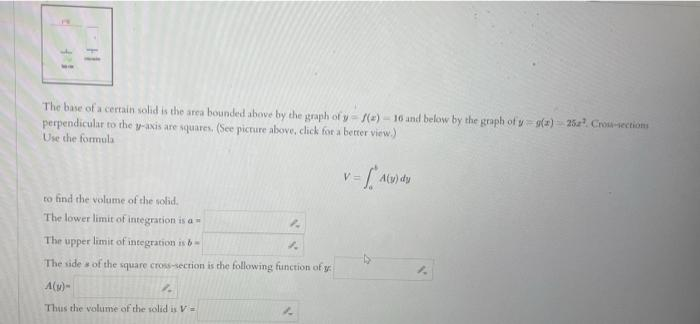# (Solved): The base of a cerrain solid is the area bounded ahove by the graph of $$y=f(x)-16$$ and below by ...The base of a cerrain solid is the area bounded ahove by the graph of $$y=f(x)-16$$ and below by the graph of $$y=9(x)=25 z^{3}$$. Crisiaectioni perpendicular to the $$y$$-axis are squares, (See picture above, click for a better virw.) Wse the formula $V=\int_{0}^{b} A(y) d y$ to find the volume of the solid. The lower limit of integration is $$a=$$ The upper limit of inegration is $$b=$$ The side * of the square cross-secrion is the following function of $$y$$ $A(v)=$ Thus the volume of the solid is $$V=$$

We have an Answer from Expert